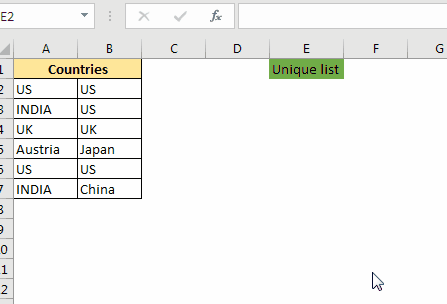# Extract Unique Values in Excel using one functionIn our previous articles, we have learned how to extract unique values from a range using a combination of different excel functions. Although they work fantastically but they are complex too, there's no denying this. If you do the extraction of unique values frequently, those formulas may tire you. They too make the file heavy and slow.

So, in this article, we will learn how to create a user-defined function that takes a range as an argument and returns only unique values from that range. You can directly copy the code to your file and start using it immediately.

VBA code for unique Function:

```Function UNIQUES(rng As Range) As Variant()
Dim list As New Collection
Dim Ulist() As Variant

'Adding each value of rng to the collection.
On Error Resume Next
For Each Value In rng
'here value and key are the same. The collection does not allow duplicate keys hence only unique values will remain.
Next
On Error GoTo 0

'Defining the length of the array to the number of unique values. Since the array starts from 0, we subtract 1.
ReDim Ulist(list.Count - 1, 0)

'Adding unique value to the array.
For i = 0 To list.Count - 1
Ulist(i, 0) = list(i + 1)
Next

'Printing the array
UNIQUES = Ulist
End Function
```

Copy the code to VB editor of excel.

Using the UNIQUE function

The above function is a user-defined multi-cell array function. It means that you have to select the range where you want the unique list to be printed then write the formula and hit the CTRL+SHIFT+ENTER key combination.

In the gif above, we have a list of countries. Now there are many duplicate countries on the list. We want to get the list of unique countries only.

To do so, select a range where you want the unique list. Now write this formula:

 =UNIQUES(A2:B7)

Hit CTRL+SHIFT+ENTER key combination. And it is done. All unique values are listed in the selected range.

Note: If the range you have selected is more than the unique value, then they will show #N/A error. You can use it as an indication of ending unique values. If you don't see #N/A at the end of the list, it means there may be more unique values.

Explanation of code

I have used two major concepts of VBA in this UD function. Collections and User Defined Array Function.  First, we have used a collection to get the unique values from the provided range.

```for Each Value In rng
next
```

Since we can't print a collection on the sheet, we transferred it to another array UList.

```for i = 0 To list.Count - 1
Ulist(i, 0) = list(i + 1) Next
next
```

Note: VBA array indexing starts from 0 and Collection indexing starts from 1. This is why we have subtracted 1 for array in for loop and added 1 to the indexing of list collection.

In the end, we have printed that array to the sheet.

There's a UNIQUE function that is not available to excel 2016 that does the same thing. That function is available in Excel 2019. Only the members of the insider's program have access to that function. By using this technique, you can show off in the office for being ahead of MS.

So yeah guys, this how you can create a function that extracts unique values simply. I hope I was explanatory enough to make you understand this. If you have any specific questions regarding this or any other excel VBA related question, ask it in the comments section below.

Related Articles:

How to use VBA Collections in Excel | Learn use of collection that can help you get unique values.

Create VBA Function to Return Array | Learn how to create a user-defined array function that returns an array.

Arrays in Excel Formul|Learn what arrays are in excel.

How to Create User Defined Function through VBA | Learn how to create user-defined functions in Excel

Using a User Defined Function (UDF) from another workbook using VBA in Microsoft Excel | Use the user-defined function in another workbook of Excel

Return error values from user-defined functions using VBA in Microsoft Excel | Learn how you can return error values from a user-defined function

Terms and Conditions of use

The applications/code on this site are distributed as is and without warranties or liability. In no event shall the owner of the copyrights, or the authors of the applications/code be liable for any loss of profit, any problems or any damage resulting from the use or evaluation of the applications/code.